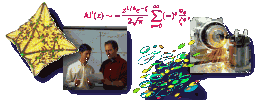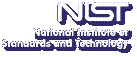Up# The Painleve equations-nonlinear special functions.

Peter Clarkson
Institute of Mathematics and Statistics, University of Kent, UK

Tuesday, November 20, 2001 11:00-12:00,
Room 145, NIST North (820)
Gaithersburg
Tuesday, November 20, 2001 09:00-10:00,
Room 5000
Boulder

Abstract: The six Painleve equations (PI-PVI) were first derived around the turn of the century in an investigation by Painleve and his colleagues in a study of nonlinear second-order ordinary differential equations. There has been considerable interest in Painleve equations over the last few years primarily due to the fact that they arise as reductions of soliton equations solvable by inverse scattering. Further, the Painleve equations are regarded as completely integrable equations and possess solutions which can be expressed in terms of the solutions of linear integral equations. Although first discovered from strictly mathematical considerations, the Painleve equations have appeared in several important physical applications including statistical mechanics, plasma physics, nonlinear waves, quantum gravity, quantum field theory, general relativity, nonlinear optics and fibre optics. The Painleve equations may also be thought of as nonlinear analogues of the classical special functions such as Bessel functions. They possess hierarchies of rational solutions and one-parameter families of solutions expressible in terms of the classical special functions for speical values of the parameters. For example, there exist solutions of PII-PVI that are expressed in terms of Airy, Bessel, parabolic cylinder, Whittaker and hypergeometric functions, respectively. Further the Painleve equations admit symmetries under affine Weyl groups which are related to the associated Backlund transformations. In this talk I shall describe some of the remarkable properties which the Painleve equations possess (including connection formulae, Backlund transformations, associated discrete equations and hierarchies of exact solutions) and some of their applications.
Contact: D. W. Lozier

Note: Visitors from outside NIST must contact Robin Bickel; (301) 975-3668; at least 24 hours in advance.